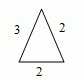Email us to get an instant 20% discount on highly effective K-12 Math & English kwizNET Programs!

#### Online Quiz (WorksheetABCD)

Questions Per Quiz = 2 4 6 8 10

### MEAP Preparation - Grade 7 Mathematics4.13 Relation Between Sides and Angles of a Triangle

 Points to remember: The sum of the measures of any two sides of a triangle is greater than the measure of the third side. The difference of the measures of any two sides of a triangle is less than the measure of the third side. If two sides of a triangle are unequal, the measure of the angle opposite to the longer side is greater than the measure of the angle opposite to the shorter side. If two angles of a triangles are unequal, then the side opposite to the greater angles is longer than the side opposite to the smaller angle. No two angles of a scalene triangle are congruent. Directions: Read the above review points carefully and answer the following questions: Illustrate each of the above review points by using the triangle given below.Illustrate each of the above review points by drawing a triangle of your own. Explain in your own words the relation between the sides and angles of a triangle with examples.
 Q 1: If BC = 10 cm and CA = 15 cm are the sides ofABC, then the measure of AB is greater than 5 cm.FalseTrue Q 2: If, inABC, ĐA = 100° and ĐB = 50°, then a < b.TrueFalse Q 3: If, inABC, ĐB = 100°, then AC is the longest side.FalseTrue Q 4: If, inABC, ĐA = 30° and ĐB = 60°, then AB is the longest side.FalseTrue Q 5: If, inABC, AB = AC, then ĐC = ĐB.TrueFalse Q 6: If, inABC, AB = 8 cm, AC = 6 cm, then BC is less than 2 cm.TrueFalse Q 7: If, inABC, ĐC = 40°, then AB is the shortest side.TrueFalse Q 8: If, inABC, AB > BC, then ĐA < ĐB.FalseTrue Question 9: This question is available to subscribers only! Question 10: This question is available to subscribers only!